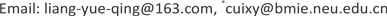1. 引言

2. 双边滤波算法

ϕ ¯ ( m 0 ¯ ) = 1 k ( m 0 ) ∑ m ∈ F ϕ ( m ) ⋅ s ( ϕ ( m 0 ) , ϕ ( m ) ) ⋅ c ( m 0 , m ) (1)

s ( ϕ ( m 0 ) , ϕ ( m ) ) = e − 1 2 ( ‖ ϕ ( m 0 ) − ϕ ( m ) ‖ σ p h ) 2 (2)

c ( m 0 , m ) = e − 1 2 ( ‖ m 0 − m ‖ σ c ) 2 (3)

k ( m 0 ) = ∑ m ∈ F S ( ϕ ( m 0 ) , ϕ ( m ) ) ⋅ c ( m 0 , m ) (4)

3. 双边滤波算法的FPGA实现3.1. 双边滤波处理步骤

3.2. 亮度相似度权重系数计算

1) 像素值的位宽N；

2) 参数σph

3) 权重系数的位宽W；

3.3. 空间邻近度权重系数计算

3.4. 归一化

4. 实验结果

5. 结论